Trial ends in

# Solutions and Concentrations

### Overview

Source: Laboratory of Dr. Michael Evans — Georgia Institute of Technology

A solution is a homogeneous mixture containing some components in small amounts, called solutes, and one component in a large amount, called the solvent. Solid-liquid solutions contain one or more solid solutes dissolved in a liquid solvent. Solutions are ubiquitous in chemistry: they are used to store and handle small amounts of material, carry out chemical reactions, and develop materials with controllable properties.

The density of a solute in a solution is known as the concentration of the solute. Concentration can be expressed in several ways, differing in the units used to convey the amounts of solute, solvent, and solution.

This demonstration illustrates how to prepare a sucrose solution with a target concentration using precise analytical techniques. Additionally, various measures of the concentration of this solution are presented and explained.

### Principles

When immersed in water, many solids break apart into particles (molecules or ions) surrounded by water molecules. This process of dissolution converts a heterogeneous mixture of solid and liquid into a single homogeneous mixture consisting of liquid water and dissolved solute particles. The dissolution process for sucrose can be written as a chemical equation using the solid and aqueous phase designators. The (aq) designator following a species implies that water molecules are surrounding and solvating that species.Different solutions may contain different numbers of dissolved particles, and concentration is a measure that quantifies the density of solute particles within a solution. One fundamental measure of concentration is the mole fraction (x) of the solute: the number of moles of solute particles (nsolute) divided by the total number of moles of solution components (all solutes and solvent).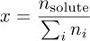Multiplying the mole fraction by 106 gives the parts per million (ppm) concentration, the number of solute particles per million particles of solution. The number of moles of solute per liter of solution, or molarity (M), is a second common measure of concentration.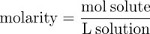Concentration may also be expressed as parts by mass, the fraction of the solution mass due to the solute.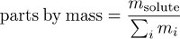Multiplying the parts by mass concentration by 100% gives the mass percent.

Finally, molality is a measure of concentration that uses the mass of the solvent, rather than the volume of the solution, as a measure of the “size” of the solution. Molality is the ratio of the number of moles of solute to the mass of the solvent in kilograms.Precise and accurate preparation of a solution with a target molarity requires careful analytical technique. The solid solute must be carefully weighed and transferred quantitatively (completely) to a volumetric flask. The solvent can then be added carefully until the solution reaches the mark on the glassware. For best results, the solute should be allowed to dissolve completely in less than the total volume of solvent, and any remaining solvent should be added when no solid solute is visible.

### Procedure

1. Preparation of 100 mL of a 0.0100 M Sucrose Solution

1. Determine the number of moles and mass of sucrose (C12H22O11) to be dissolved in 100 mL of solution.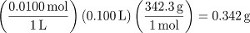2. Weigh out the mass of sucrose on the balance. First place a weigh boat on the balance and set the “tare weight”. Then using a scoopula, carefully transfer solid solute from the reagent bottle to the weigh boat until the desired amount is obtained.
3. Place a powder funnel into a clean and dry 100-mL volumetric flask. Pour the solid from the weigh boat through the funnel into the flask.
4. Using a wash bottle containing distilled water (the solvent), rinse any remaining solid from the weigh boat through the funnel into the flask.
5. Add solvent using the distilled water faucet until the liquid level reaches the neck of the flask (but not the mark). Cap and swirl the flask gently to dissolve the solute.
6. Once all of the solute has dissolved, use a wash bottle to carefully add solvent until the liquid level reaches the mark.
7. Cap and invert the volumetric flask several times to ensure good mixing of the solution.

2. Making a Supersaturated Sucrose Solution

1. Add 100 mL of distilled water to a 600-mL beaker.
2. Add 220 g of sucrose to the beaker.
3. Place a magnetic stir bar in the beaker and allow the mixture to stir for 15 min.
4. Examine the mixture: not all of the sucrose has dissolved. Heat the mixture to 50 ºC and stir for an additional 10 min.
5. Examine the mixture: all of the sucrose has dissolved at 50 ºC.
6. Allow the solution to cool to room temperature. Examine the solution: the additional sucrose that dissolved at 50 ºC remains dissolved at room temperature. The solution at room temperature is supersaturated.

Solutions are ubiquitous in chemistry. They are used to store and handle small amounts of material, carry out chemical reactions, and develop materials with controllable properties. A solution is a homogeneous mixture containing some components in small amounts, called solutes, and one component in a large amount, called the solvent. The amount of solute relative to the total amount of a solution is known as its "concentration". Depending on whether it is the mass, volume, or amount in moles of the solution components that are being considered, this measurement can be expressed in a number of different ways, according to the needs of the experiment. In this video, we will first review the different types of units for measuring a solution's concentration. We will then go through a protocol for making a sucrose solution. Finally, we'll look at how concentration measurement is used in diverse chemical applications.

The concentration of a solution can be expressed in a number of different units, each of which may be more suitable for particular applications than others. One of the most commonly used units is molarity, which is the amount of solute per volume of solution; one molar is equivalent to one mole of solute per liter of solution. Due to the simplicity of measuring the volumes of liquids, molarity is one of the most convenient units for stoichiometric calculations of reactions in solution. Stoichiometry is based on the number of molecules involved in a reaction. Therefore, knowing the molarity simplifies the calculation of required reagents.

When concentration is expressed as the amount of solute per mass of solvent, the measurement is called molality. The volume of materials changes with temperature, so measuring concentration with molality is advantageous when studying physical properties of solutions, known as colligative properties, which involve temperature differences. Mole fraction is another common concentration unit, and is given by the number of moles of solute per total number of moles of all solution components — solutes and solvent. Mole fractions are useful, for example, when investigating the "vapor pressure" of solutions. This reflects the extent to which solute and solvent particles "escape" from a liquid solution into the gaseous phase, as the mole fraction is equal to the ratio of partial pressures to total pressure. Now that you have an idea of how the concentration of a solution can be measured, let's go through a protocol for making a solution with a specific molar concentration.

Begin by calculating the mass of sucrose needed, by first using the desired volume and concentration of the solution to arrive at the number of moles of sucrose, and then using the molecular mass to convert to mass. In this example, 100 mL of a 0.01 M sucrose solution is being made, so 0.342 g will be needed. To weigh out the required mass of sucrose, first place a clean, empty weigh boat on the balance. Set the "tare weight", which means setting the weight of the empty weigh boat as zero. Then, using a scoopula, transfer the sucrose powder from the reagent bottle onto the weigh boat until the desired amount is obtained. Place a powder funnel into a clean, dry 100-mL volumetric flask. Carefully pour the sucrose through the funnel. Using a wash bottle containing the solvent, in this case distilled water, rinse any remaining solid from the weigh boat into the flask.

Add more distilled water, but stop before it reaches the calibration mark. Cap and swirl gently to dissolve the solid. It is important not to fill the flask all the way at this point, as it may be difficult for the solid to fully dissolve.

Once all the sucrose has dissolved, carefully add the solvent using a wash bottle until the bottom of the meniscus reaches the volumetric graduation. Cap the flask again and invert it several times to ensure complete dissolution and mixing.

A supersaturated solution is one in which more solute is dissolved then would be expected, given the temperature or other physical properties of the solvent. The degree to which is based on the solute, solvent, and rate of cooling. Supersaturation is achieved by first dissolving the solute in a condition where the solubility is high, and then rapidly changing the solution's condition — for example, decreasing its temperature or volume — more quickly than the solute particles can come out of solution. At this point, more solute would remain in solution under the new conditions than would be possible by dissolving the solute directly under those conditions. To create a supersaturated sucrose solution, first place 100 mL of water into a beaker. Add a magnetic stir bar, then stir on a hot plate. Add 220 g of sucrose into the stirring water, and allow the sucrose mixture to stir for 15 min. After 15 min, observe that not all of the sucrose has dissolved. At this point, heat the mixture to 50 °C. Continue stirring the mixture for an additional 10 min.

Examine the solution again. All of the sucrose should have been dissolved in the 50 °C water. Now, let the solution cool slowly to room temperature and remove the stir bar. Observe that sucrose still remains dissolved. The room-temperature solution is now supersaturated. Adding even a small amount of additional sucrose powder into this solution can trigger rapid recrystallization of all the dissolved sucrose.

Now that you have seen how to prepare solutions with specific concentrations, let's look at a few examples of how the concept can be an important consideration for various applications.

Concentration of reagents, solvent components, and other components of a chemical reaction often have significant impact on the rate of products of the reaction. Higher reactant concentrations increase the likelihood that the molecules will encounter each other and react, thus potentially increasing the reaction rate. At the same time, increased concentrations of charged salt ions in solution may also favor the aggregation of hydrophobic, or "water-repelling" molecules.

Researchers here studied the self-assembly of a complex molecule into long polymers in the presence of varying concentrations of salt in the reaction solvent. They found that, at higher salt concentrations, assembly of the molecules into polymers occurs more readily.

Concentration also affects the rate of physical processes such as crystallization. Biologists often crystalize molecules such as proteins, where they become neatly arranged in a crystal lattice, so their structure can be deduced by studying how X-ray diffracts through these crystals. To crystalize proteins, protein solutions are mixed with a "precipitant", usually a salt of some kind, at different concentrations and pH. A drop of this mixture is then placed into a closed chamber with a reservoir of more concentrated precipitant solution. As water evaporates from the protein solution droplet in order to equilibrate the precipitant concentration between the droplet and the reservoir, the protein becomes increasingly supersaturated and eventually crystallizes out of the solution. For more information, see our video on growing crystals.

Finally, an understanding of concentration is important for evaluating levels of toxins in the environment. In this example, scientists developed an assay to detect the amount of the potentially fatal bacterial toxin botulinum in food or water samples, by detecting the extent to which the toxin cleaves a particular protein. To perform the assay, a "standard curve" is first generated by measuring the activity level of different known concentrations of the toxin. Toxin isolated from unknown samples can then be subjected to the test, and the concentration can be interpolated by comparing its activity to the standard curve.

You've just watched JoVE's introduction to making solutions. You should now understand when to use different units for expressing concentration, a demonstration for making a solution with a specific concentration, and finally, several applications that illustrate the topic's importance.

Thanks for watching!

### Results

Procedure step 1 creates 100 mL of a 0.0100 M sucrose solution. To convert to measures of concentration other than molarity, determine the mass of water used to prepare the solution. Although this can be measured accurately, in the absence of a measurement it can be assumed that the volume of dissolved solute particles is negligible (i.e., the volume of water used was 100 mL). Using the density of water…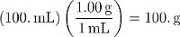The molality of sucrose in this solution is thus: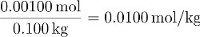The parts by mass of sucrose is equal to: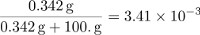The mole fraction of sucrose can be calculated by determining the number of moles in 100 g of water and dividing the amount of sucrose by the total amount of particles in the solution.Procedure step 2 illustrates that the solubility of sucrose in water is temperature dependent. Upon heating, undissolved sucrose resting in a saturated solution dissolves, forming a saturated solution of higher concentration at higher temperature. When this solution cools, sucrose does not precipitate out of the solution. The resulting cooled solution is supersaturated with sucrose. Adding even a small amount of additional sucrose powder into this solution can trigger rapid recrystallization of all the dissolved sucrose.

### Applications and Summary

Solid-liquid solutions are ubiquitous in chemistry. Most chemical reactions are run in solution because dissolved solutes are mobile enough to rapidly mix and bump into one another. Solutions can also be used to store small amounts of solutes in macroscopic and easily handled volumes. Solutions exhibit some interesting physical properties called colligative properties that can be attributed to the entropic effects of dissolving a solute in a solvent.

One may wonder why so many different measures of solution concentration exist. The answer lies in the many applications of solutions and the many orders of magnitude over which concentrations span. In samples of water from the environment, for example, concentrations of metal ions can be in the range of a few parts per million — it is impractical and potentially misleading to express this tiny concentration as a molarity or mole fraction. Although molarity is a convenient measure of concentration for stoichiometry calculations involving chemical reactions, molality is more appropriate in studies of certain colligative properties.

Perfecting the technique of solution preparation is important, because in many contexts, precise knowledge of concentration is essential. When running a chemical reaction, for example, use of too much or too little solute could result in wasted reactants or low product yields. Studies of empirical relationships involving concentration, such as Beer’s law, depend on precisely known concentrations. Oftentimes, imprecision in solution concentrations leads directly to uncertainty in calculated values, such as reaction enthalpies. Although it is impossible to completely eliminate imprecision, the use of analytical techniques for solution making ensures that uncertainty is minimized.X